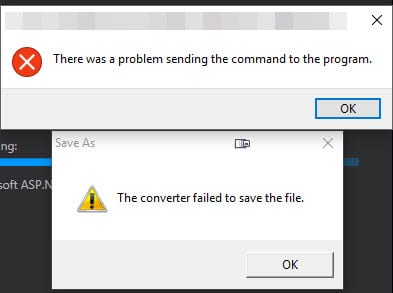After years of using Excel, I realized today I have never had to round up to the nearest whole number before – until today that is. I was organizing my finances and realized that I wanted to round some of the amounts up to the nearest 10. So to round to the nearest decimal place in Excel, the formula is:

=ROUNDUP([Range],[Position])

Count the number of places after the 0 to round to and set [Position] to that value

So if you had 1234.25 in Cell A1 and wanted to round up to the nearest cent, =ROUNDUP(A1, 1) would produce 1234.30.

 1234.25 ^ 1 1234.25 ^ 2 =ROUNDUP(A1,1) =ROUNDUP(A1,2)

Want to go the other way? Simply start at the decimal as 0 and count backwards in the negated position.

 1234.25 ^ -1 1234.25 ^ -2 1234.25 ^ -3 1234.25 ^ -4 =ROUNDUP(A1, -1) =ROUNDUP(A1, -2) =ROUNDUP(A1, -3) =ROUNDUP(A1, -4)

To round to the nearest ten (10)
=ROUNDUP(A1, -1) = 1240
To round to the nearest hundred (100)
=ROUNDUP(A1, -2) = 1300
To round to the nearest thousand (100)
=ROUNDUP(A1, -3) = 2000• ####SpreadSheetNinja

Now here comes the next problem 😛
When you relize you have to round up to a number based on a list.

example :

A1 contains number 5

and B1 to B10 contains numbers like 35, 70, 94, 120 and so on the spesific numbers are irrelevant, the point is rounding to them.

so in this example one would want 5 to be rounded up to 35. ditto if the number in A1 is 36 then it would round to 70.

Cant use vba on it seeing the bosses freaks out about virus warnings.